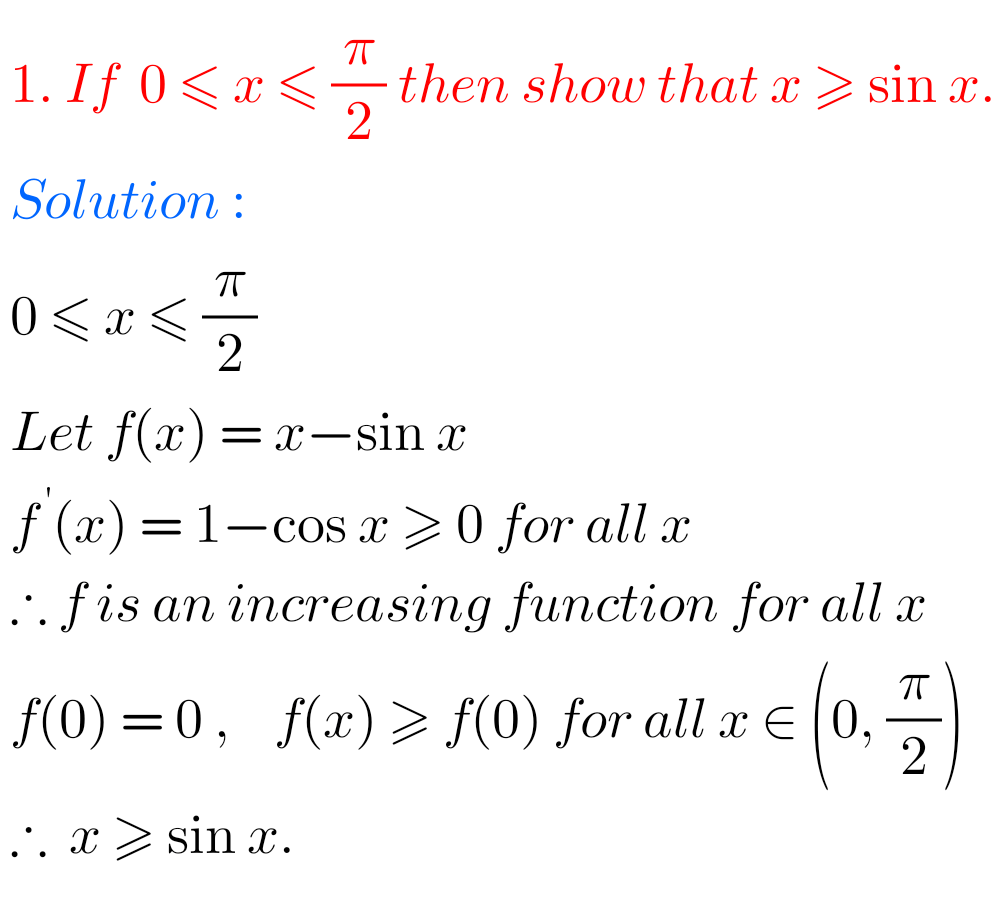# Inter Maths Solutions for Applications of Derivatives exercise 10(h)

Intermediate Mathematics 1B Applications of Derivatives exercise 10(h) textbook solutions are given.

Study the lesson Applications of Derivatives very well.

Practice the example problems and solutions given in the textbook.

Observe the given below solutions and try them in your own method

You can also see

Inter Maths 1A textbook solutions

Inter Maths 1B textbook solutions

Inter Maths IIA textbook solutions

Inter Maths IIB textbook solutions

Applications of Derivatives

Exercise 10(a)

Exercise 10(b)

Exercise 10(c)

Exercise 10(d)

Exercise 10(e)

Exercise 10(f)

Exercise 10(g)

M

## Applications of Derivatives Exercise 10(h) solutions Inter Maths

Chapter 10 – Aplications of Derivatives

Exercise 10(h)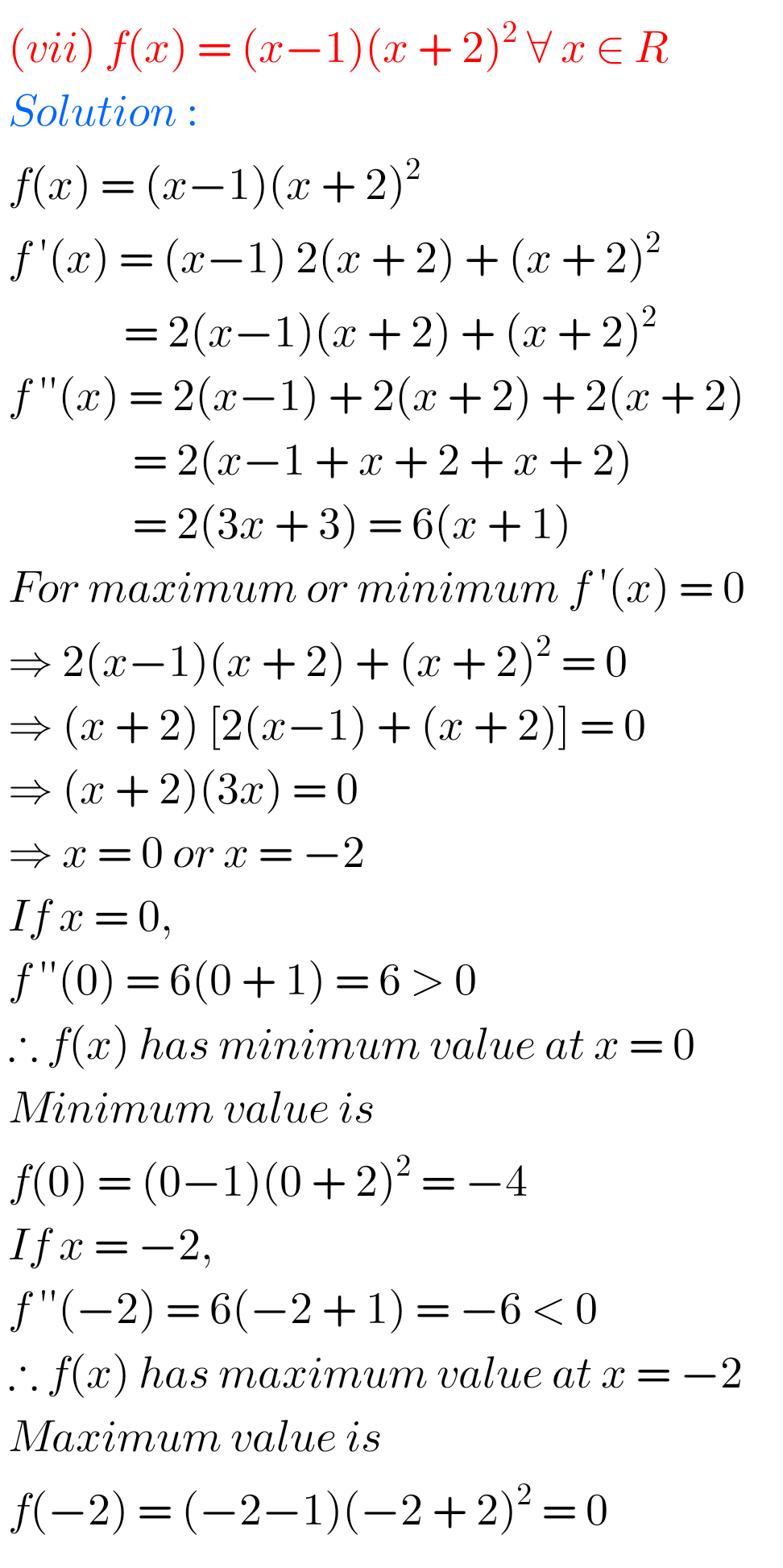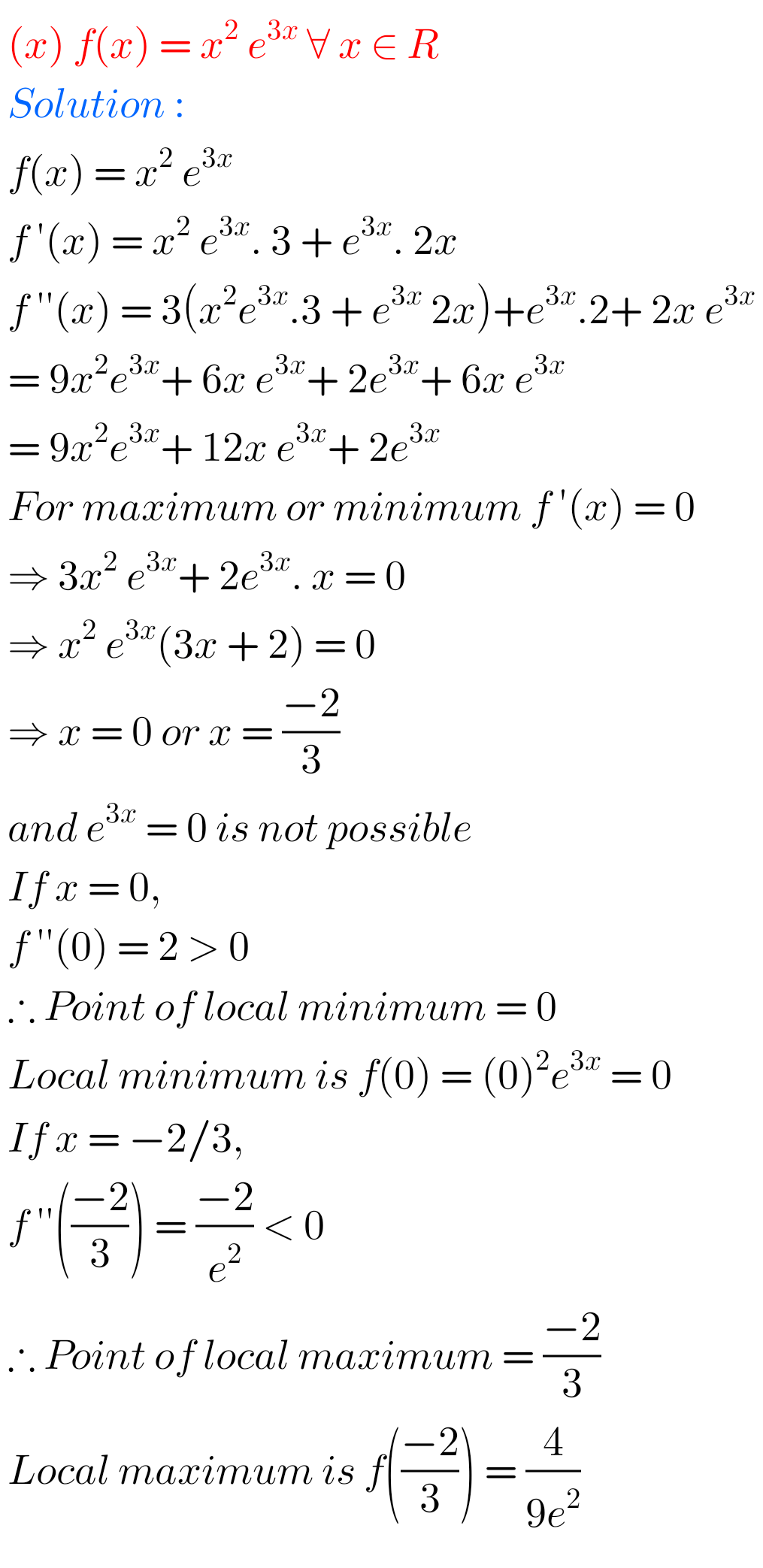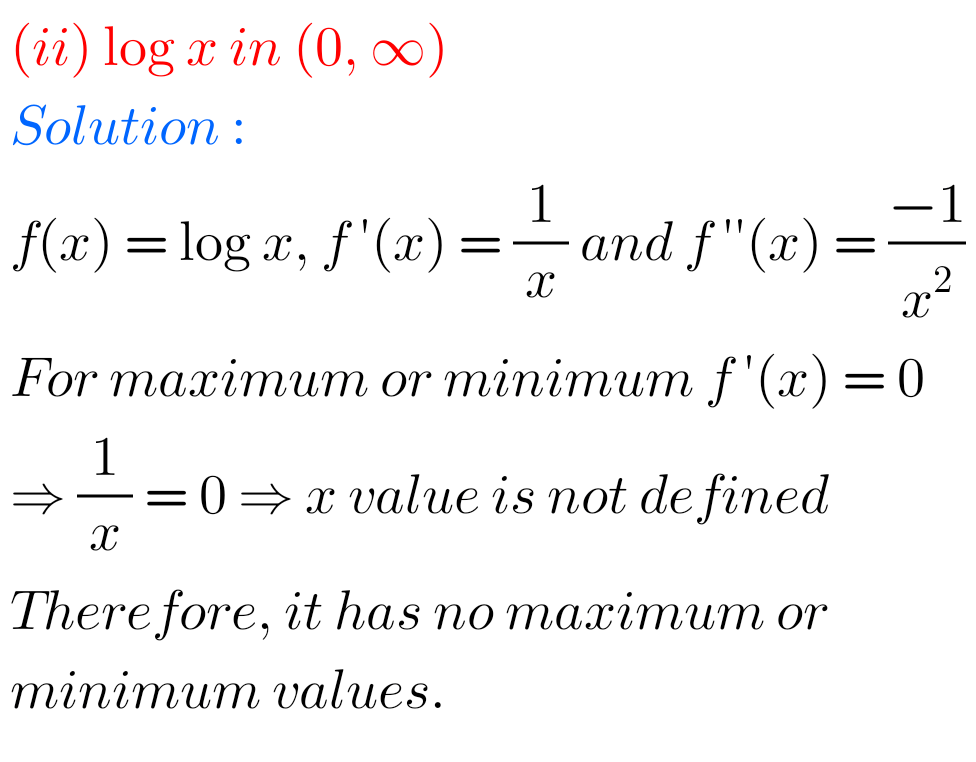## Solutions for Exercise 10(h) Applications of Derivatives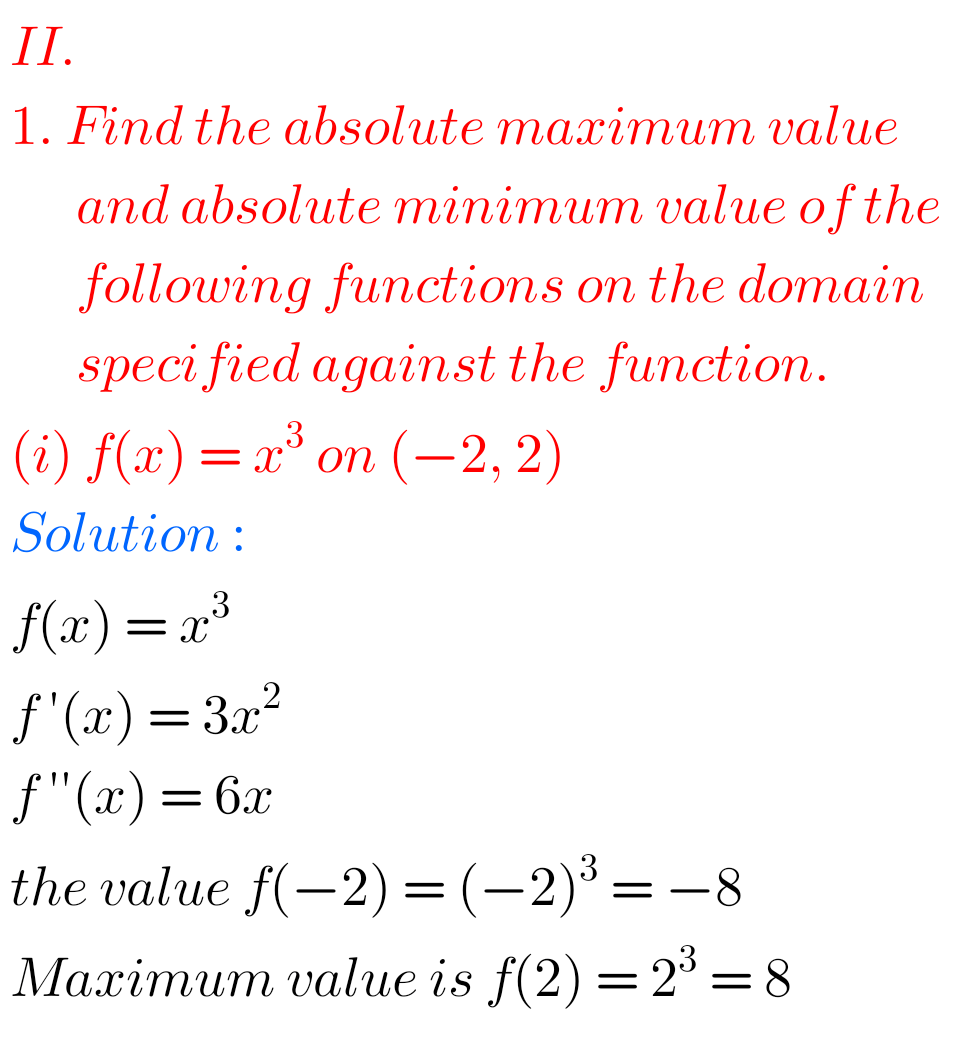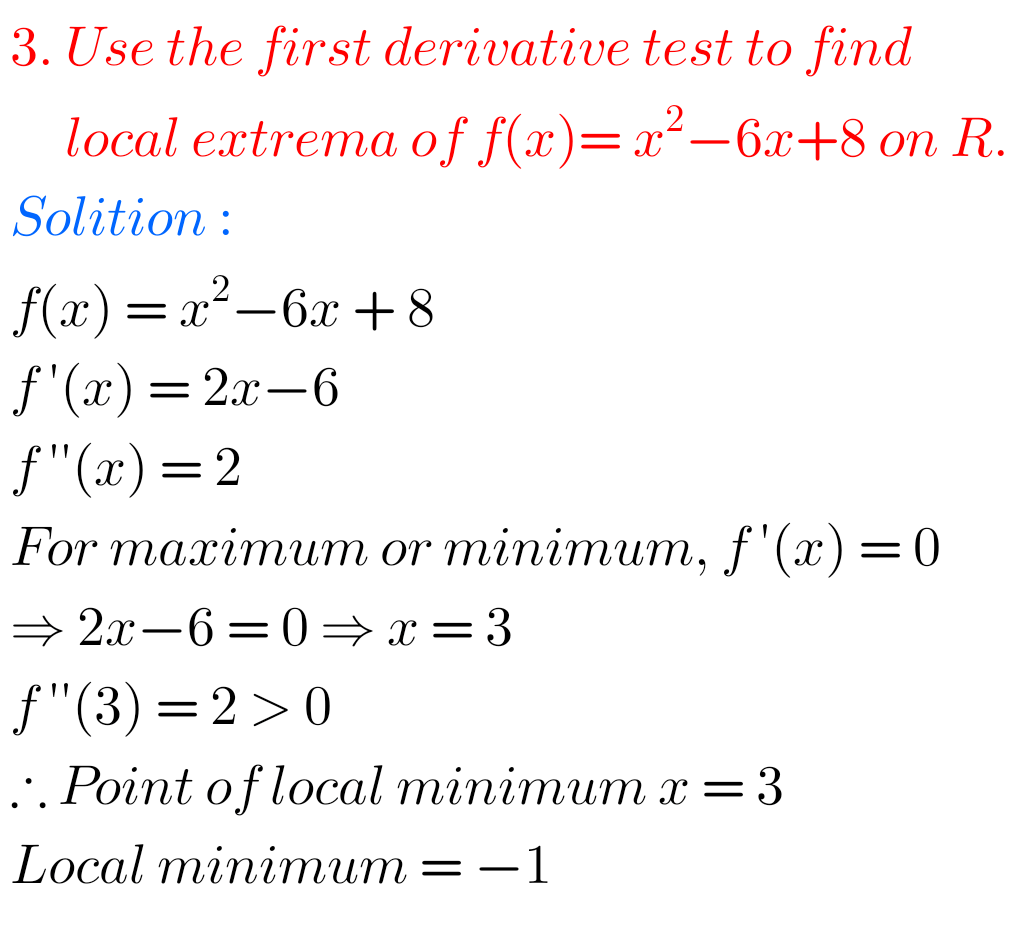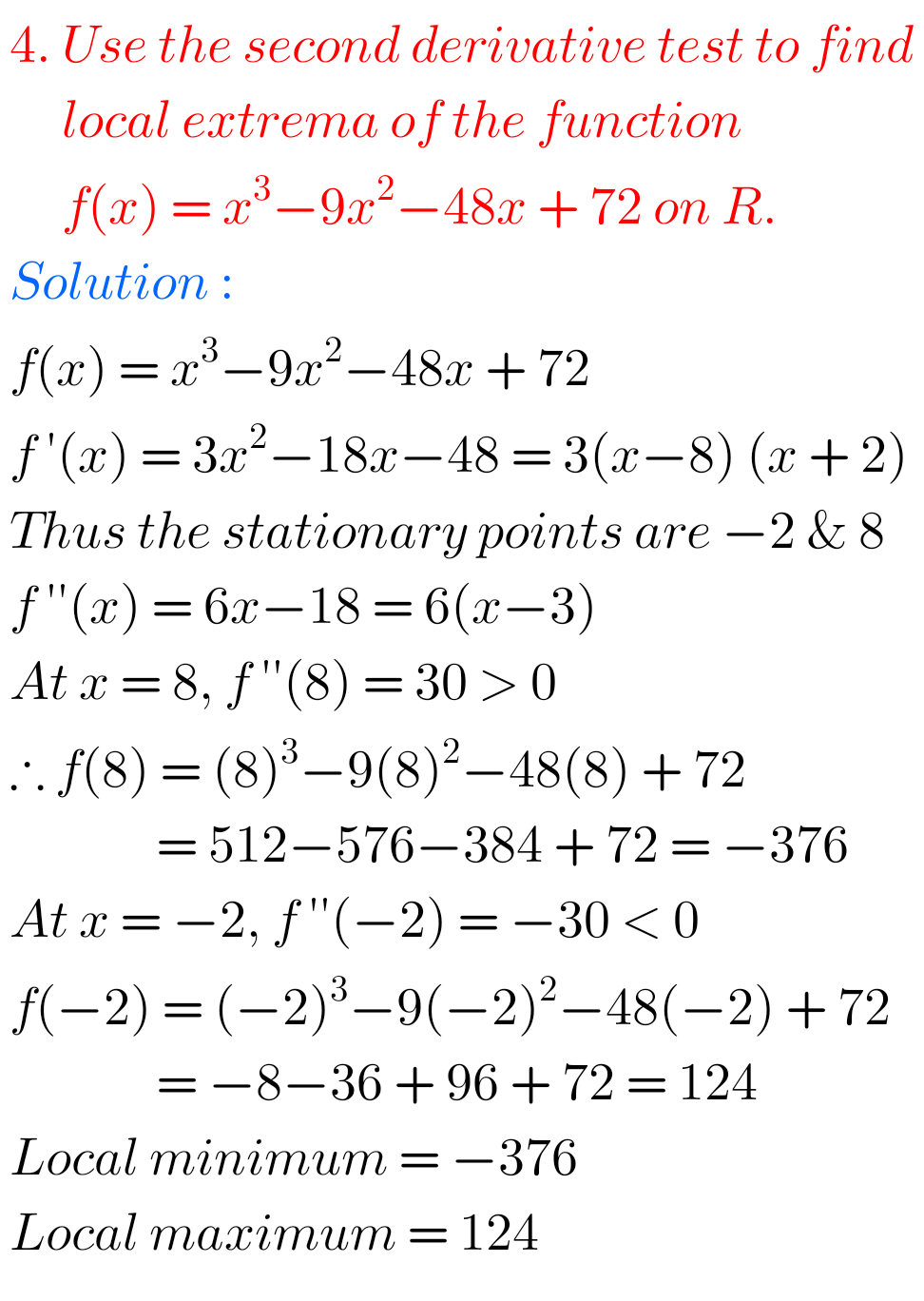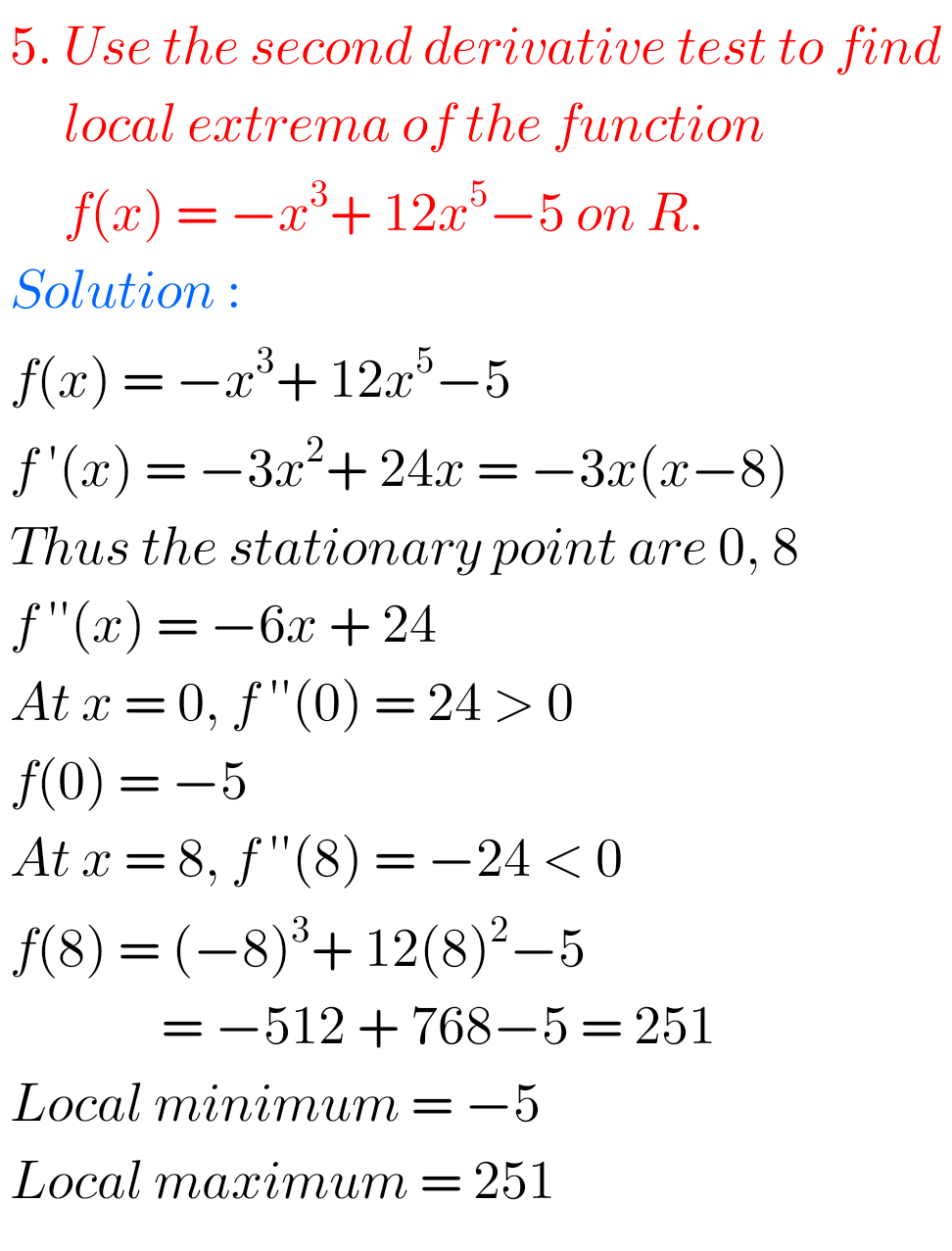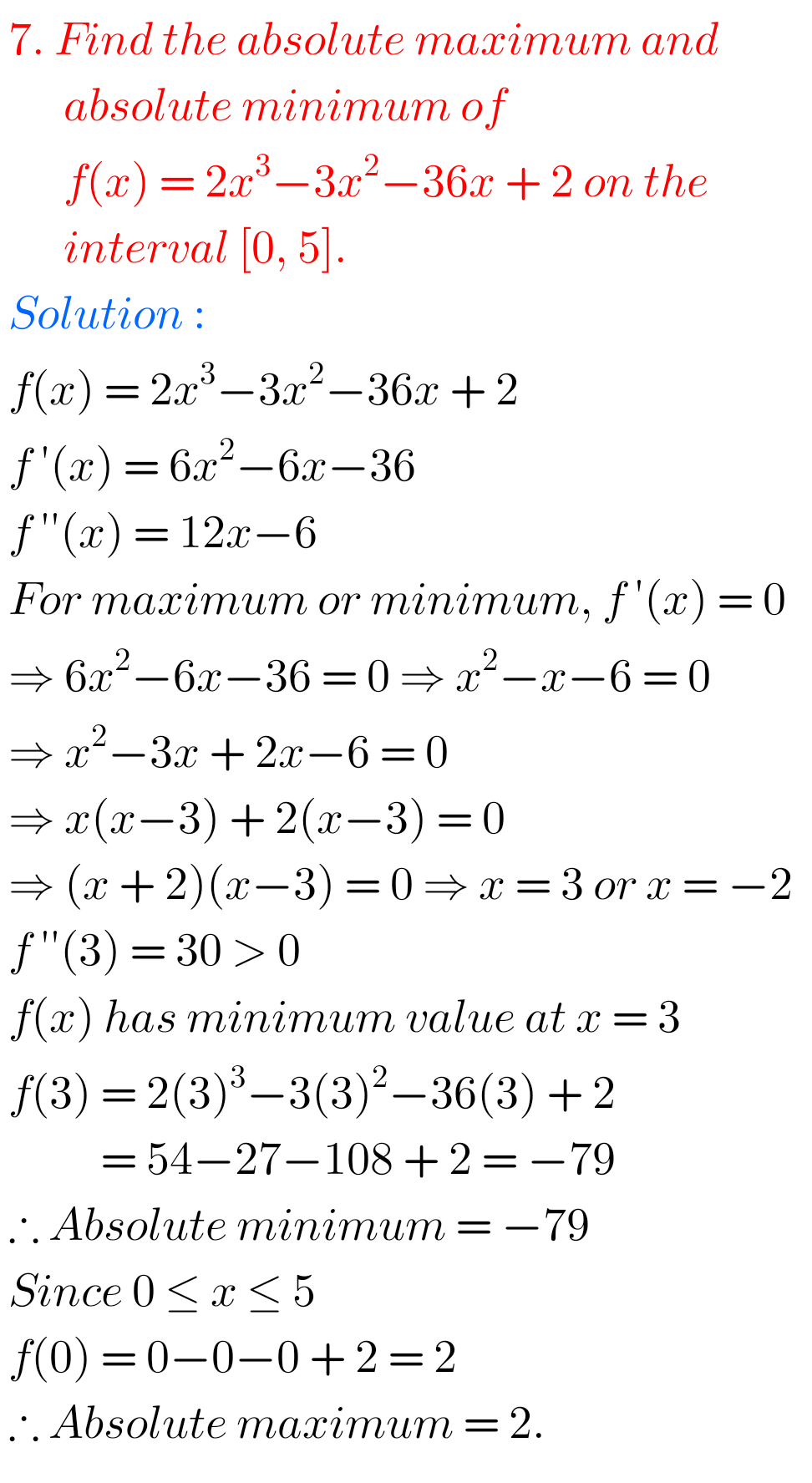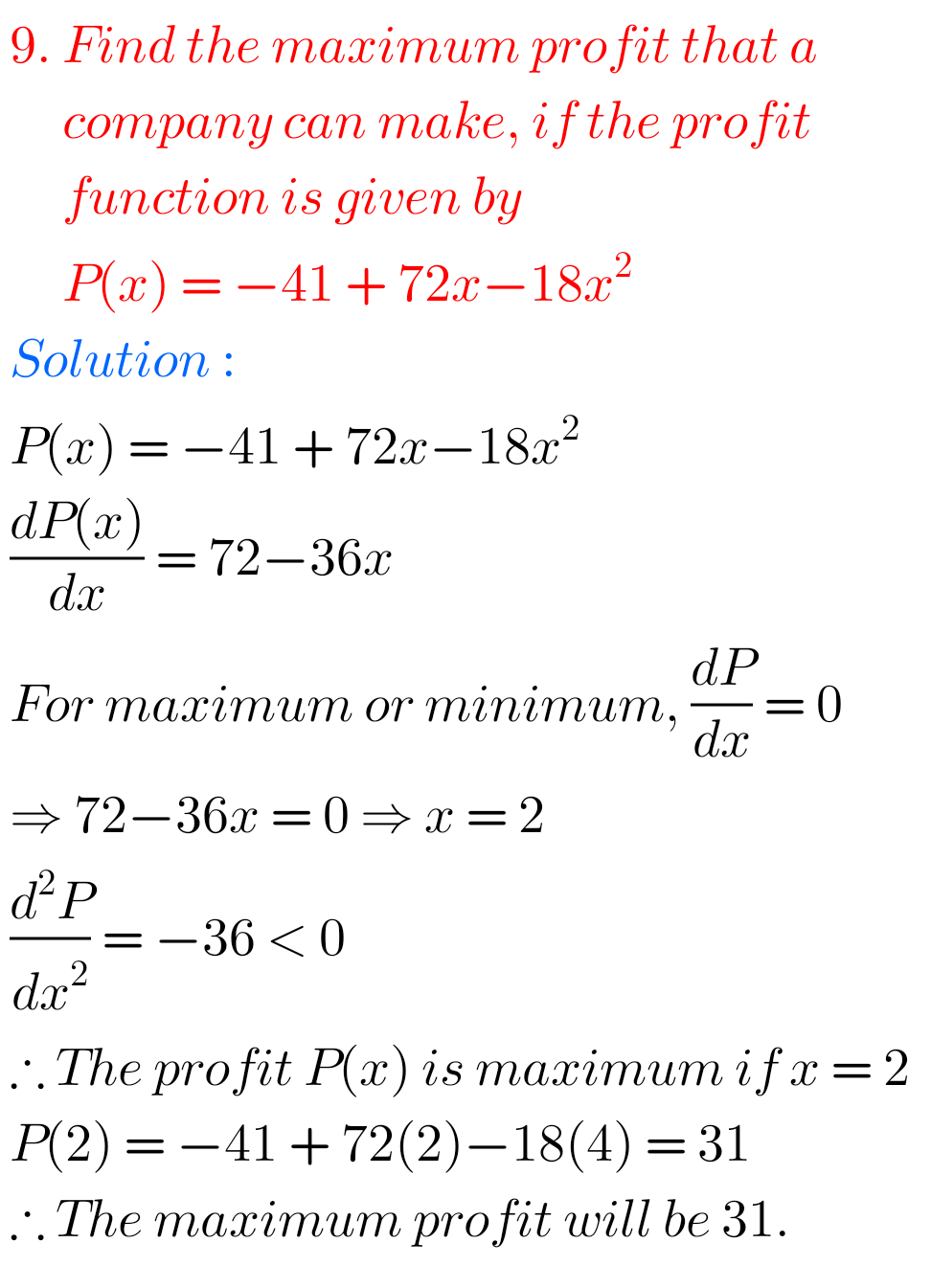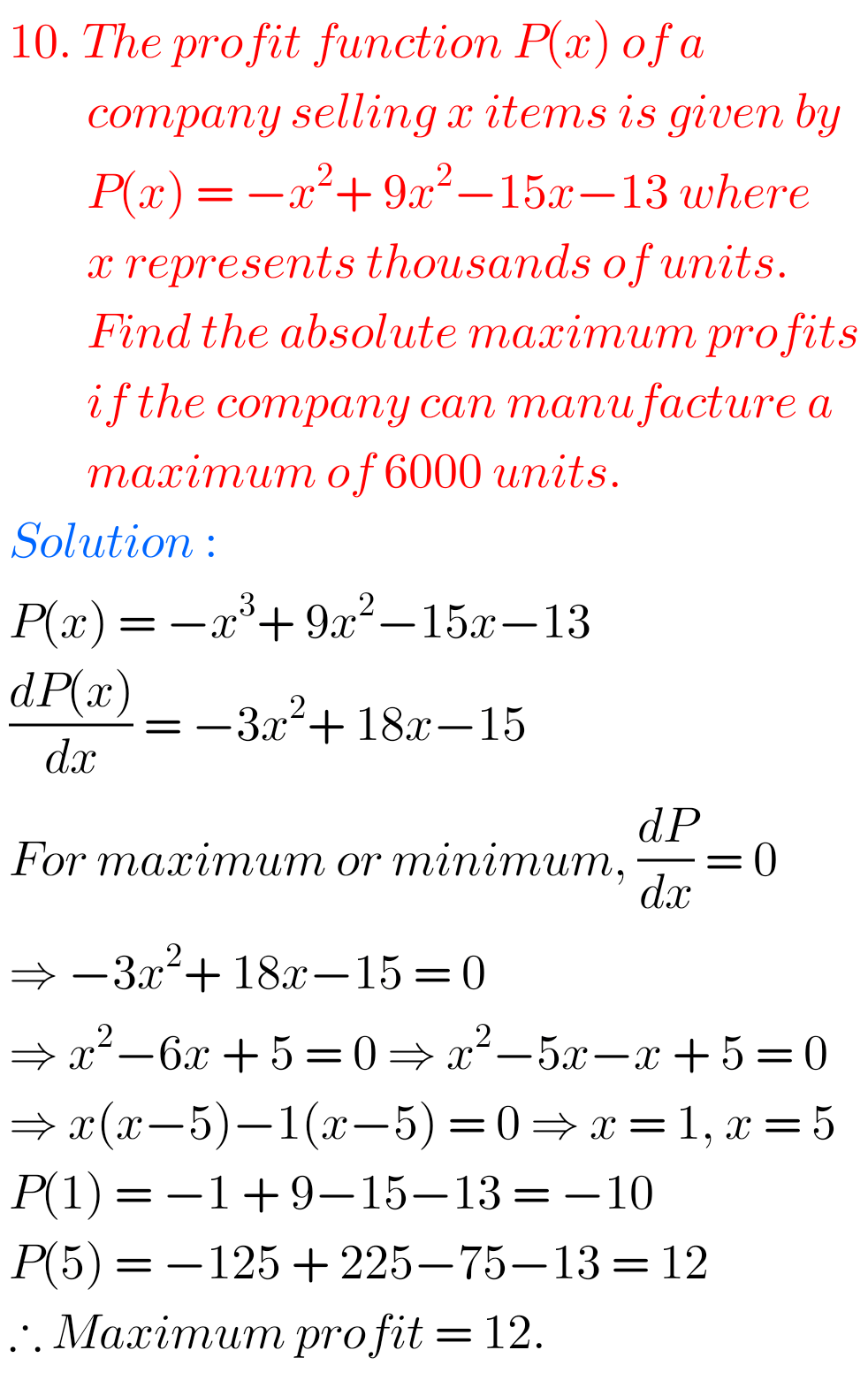## Inter 1st year Maths 1B solutions for Applications of Derivatives exercise 10(h)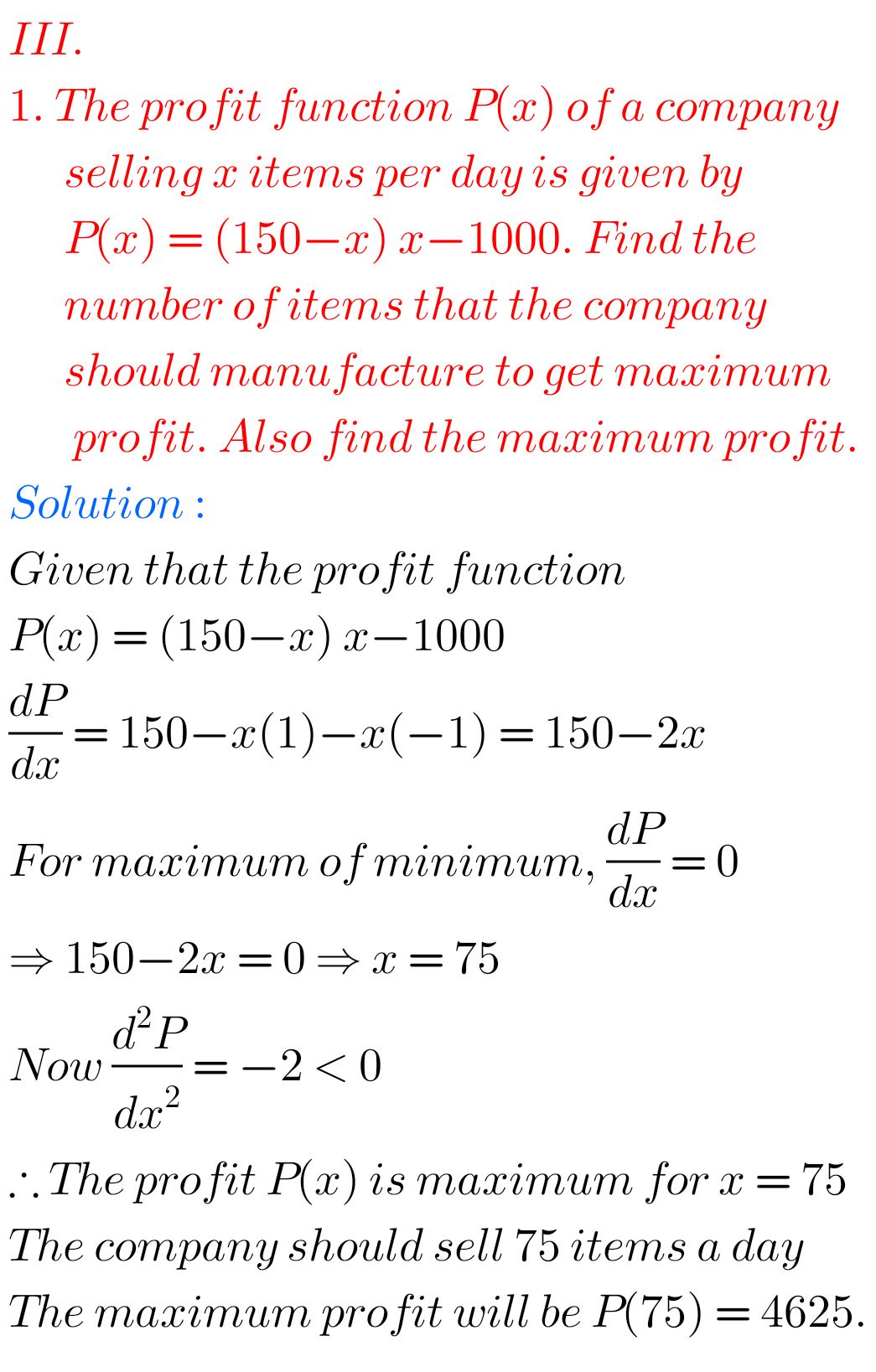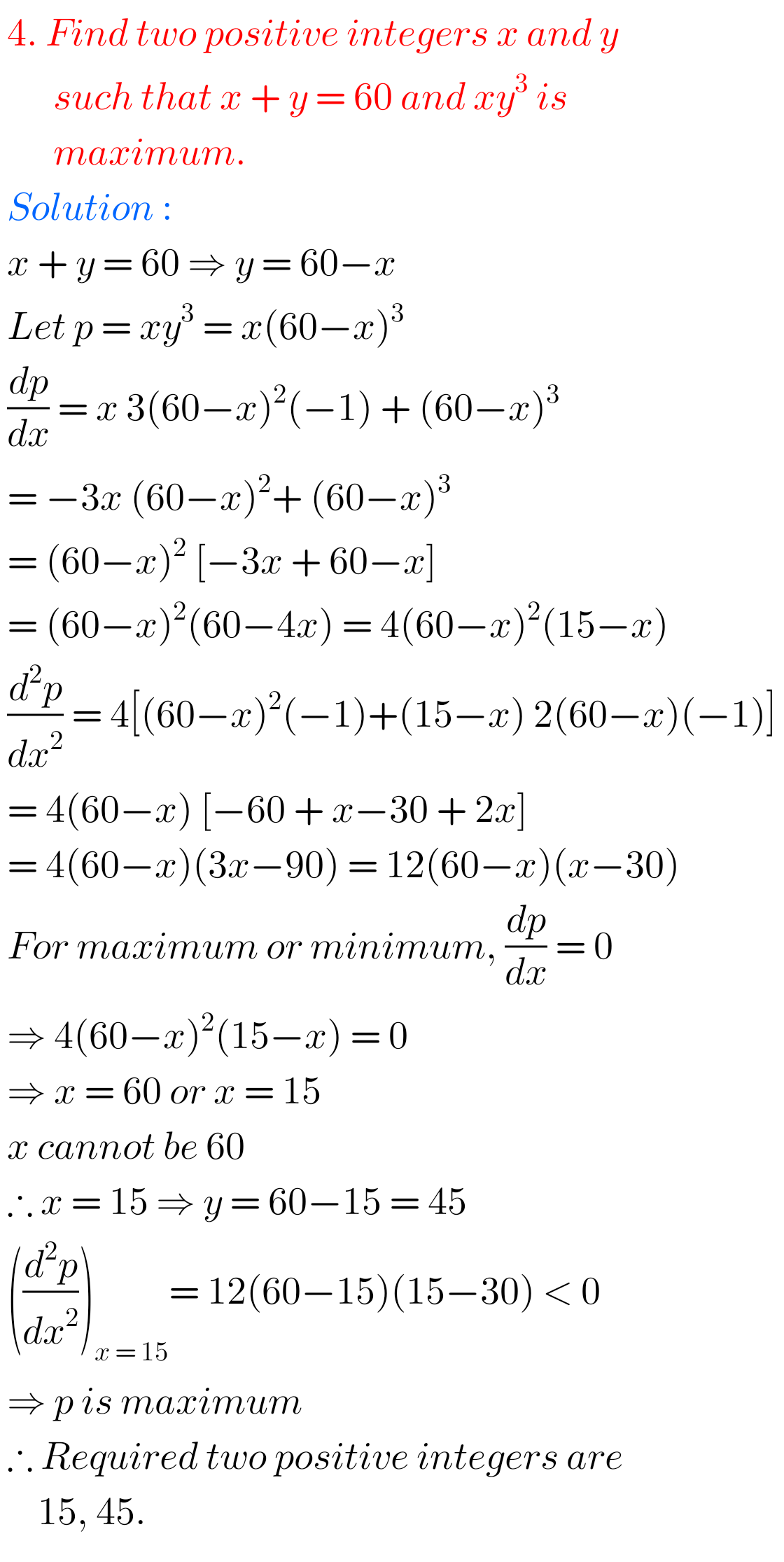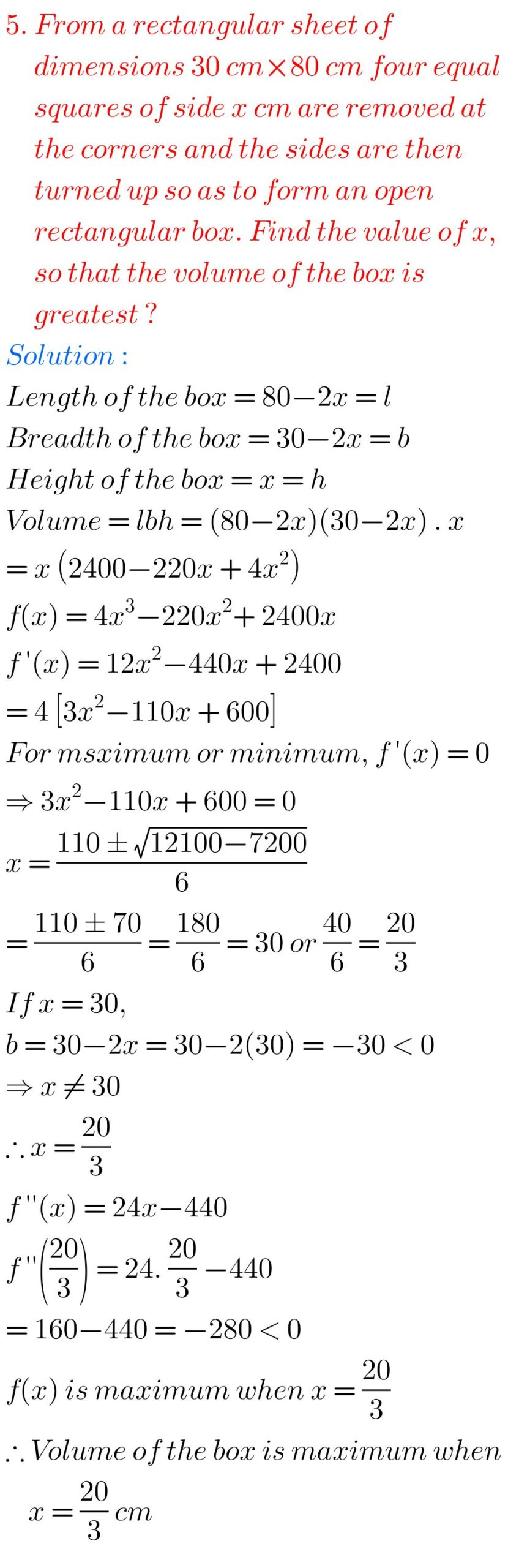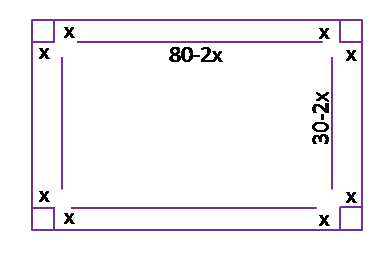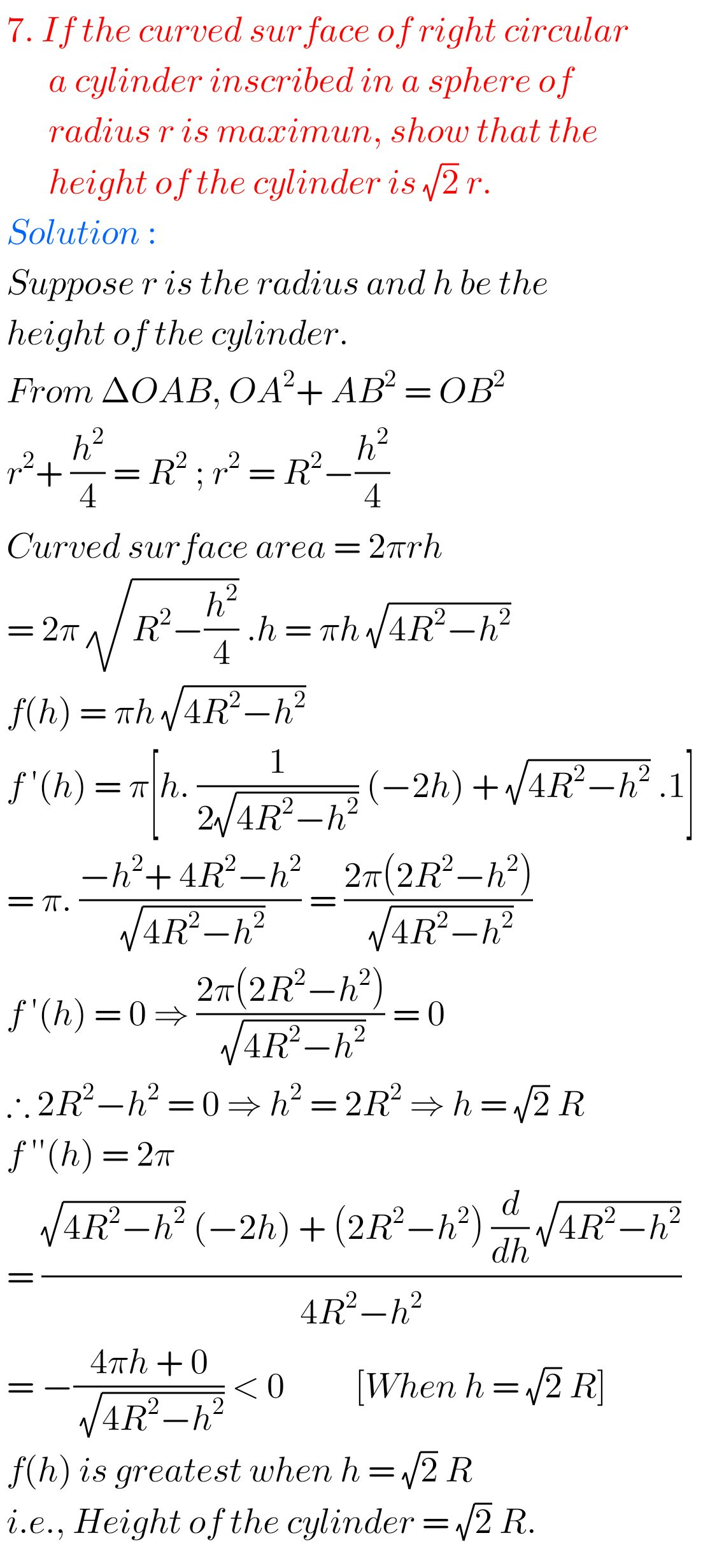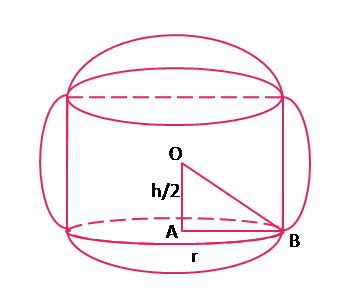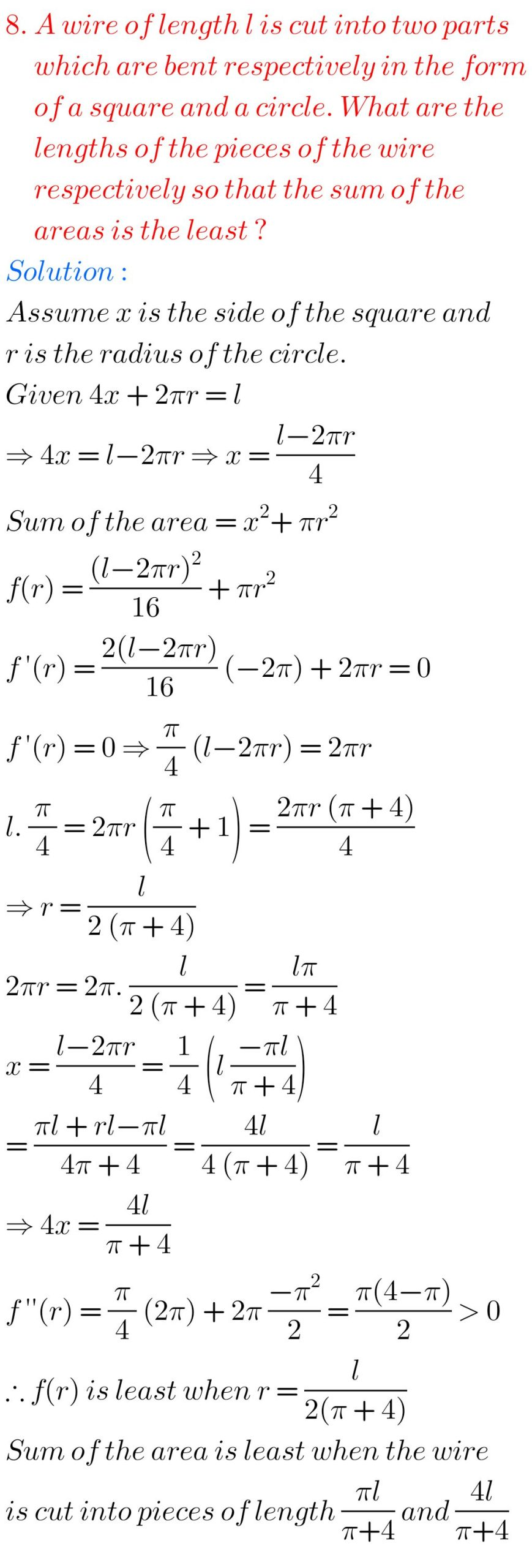Note : Observe the solutions and try them in your own method.